# Linearization Of Wheatstone-bridge

### 要約

This application note discusses the resistance-variable element in a Wheatstone bridge—the first choices for front-end sensors. We will examine its behavior and explain how to linearize the bridge circuit to optimize performance. The simplicity and effectiveness of a bridge circuit makes it very useful for monitoring temperature, mass, pressure, humidity, light, and other analog properties in industrial and medical applications.

### Introduction

Wheatstone bridge circuits measure an unknown electrical resistance by balancing two legs—one with the unknown component—of a bridge circuit. These long-established circuits are among the first choices for front-end sensors. Whether the bridges are symmetric or asymmetric, balanced or unbalanced, they allow you to accurately measure an unknown impedance. Since bridge circuit are so simple yet effective, they’re very useful for monitoring temperature, mass, pressure, humidity, light, and other analog properties in industrial and medical applications.

The Wheatstone bridge has a single impedance-variable element that, when away from the balance point, is inherently nonlinear. Bridge circuits are commonly used to detect the temperature of a boiler, chamber, or a process situated hundreds of feet away from the actual circuit. Usually a sensor element, typically a resistance temperature detector (RTD), thermistor, or thermocouple, is situated in the hot/cold environment to provide information about resistance change to temperature.

In this application note we will take a look at its behavior and explain how you can linearize the bridge circuit to enhance performance. Note that when we speak generally about “bridges,” this piece is focused on circuit design for a Wheatstone bridge.

### Wheatstone Bridge with Single Variable Resistance

Using inexpensive, accurate discrete parts, resistance-variable Wheatstone bridge circuits perform most of the front-end tasks in a design. By incorporating an RTD element (and based on the RTD manufacturer), the bridge’s inherent resistance variations stay within the accepted linearity and tolerance limits.

RTD devices generally come with very detailed data sheets characterizing their behavior with look-up tables and even transfer function equations down to four or more orders of error compensating terms. For a high-precision system, desiginers have traditionally had to consider both the inherent nonlinearity of the RTD element and the Wheatstone bridge, and then painfully calibrate the front-end while linearizing the front-end at the microcontroller side. Linearity improves by increasing the order of the equation in the microcontroller. A typical bridge circuit (Figure 1) detects milliohms of changes in resistance (ΔR).Assuming that R1 = R2 = R3 = R4 = R in Figure 1, the bridge is balanced with nodes A and B at a constant V/2 (volts) and with a differential voltage of 0V across VAB. Should a change occur in resistance (ΔR) from R3, then the output differential voltage created is:When R1 = R2 = R3 = R4 = R, the bridge is balanced.

For a single variable-resistance element, for R3 = R + ΔR and R1 = R2 = R4 = R:Equation 2 suggests that increasing the constant supply voltage, V, to the bridge will increase the output voltage, i.e., the swing range across the bridge. It’s important to note that this equation also suggests that having a dual supply across the four-legged resistance arrangement could be useful not only to increase the range, but also to help maintain a 0V common-mode voltage across the AB nodes.

The voltage VAB is usually amplified by using a subsequent amplifying stage via a differential amplifier. However, note that changing the common-mode voltage across VAB increases error and complexity in the amplifying second stage, usually realized as a instrumentation quality differential amplifier. For this reason, it’s a good idea, and easier to manage, to have, a common-mode voltage centered around 0V.

In Figure 2, you can see the natural tendency of the bridge’s single-variable element in the form of inherent nonlinearity in its transfer function.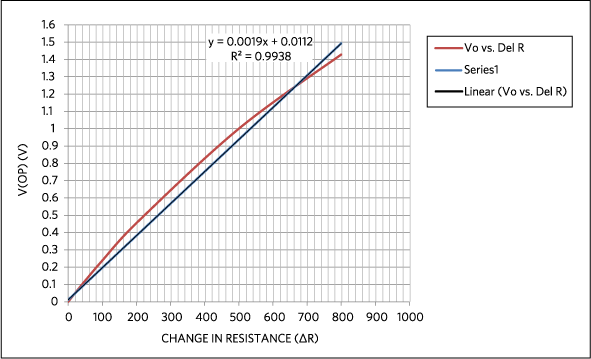In Figure 2, take a closer look at the trend line. The linearity error, or the curve’s absolute deviation from an ideal straight line, is about 0.62%. This percentage is generated by comparing the curved trend line with the line of best fit, i.e., the straight line relative to the curve. This approach quantifies the worst-case linearity error for the above curved line. In some cases, a 0.6% linear is certainly not acceptable. In this application note, we’ll examine a method to achieve better than 0.1% accuracy.

Aside from addressing the bridge’s inherent nonlinearity, you must also manage the nonlinearity of the temperature sensor element, RTD, or even thermistor as discussed previously. The instrumentation amplifier (Figure 3) has a common-mode voltage of V/2 when sensing the differential voltage across the nodes A and B. The amplifier is usually either a differential amplifier with four resistors or a three-op-amp instrumentation amplifier integrated in a single package.When a differential amplifier is used, the nodes A and B are connected to the amplifier’s input gain-setting resistors, as shown in Figure 3. The choice of the op amp and the input resistors is signficant as this path directs current away from the bridge, which impacts accuracy.

Also, the type of resistors you use affects the bridge performance. For instance, even 0.1%-tolerant resistors used with the amplifier provide only 60dB of common-mode rejection.

### Linearize the Bridge Output Without the Instrumentation Amplifier

Based on our earlier discussion, having dual supplies across the resistor bridge to increase the dynamic range seems a logical approach. It would also make sense to have the sensing nodes centered around the 0V common mode. In such a design, the transfer function from the node B will be linear with a change in resistance. Compared to the output from the circuit in Figure 1, the range of the output swing from the bridge would be doubled.

In Figure 4, we see a circuit implementation with two op amps in place of a more complex instrumentation amplifier. With this design approach, the linearized bridge output avoids the unnecessary current paths created by the differential amplifier. This presents an easier design process versus the circuit in Figure 3. However, there are positive and negative supplies to the amplifiers, providing twice the swing range. Better common-mode rejection performance is an added advantage, as the second amplifier operates comfortably around 0V.From Figure 4, node A sees GND as it is the summing node of amplifier 1. Thus, a constant current of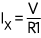is forced through the R1|R3 branch, producing an equal and opposite voltage on the other side of the bridge with -V. When the single variable-resistance R3 changes (from R3 to R ±ΔR), then Ix (the change in current due to change in the resistance) flowing through this resistance produces a voltage V ±ΔV. A factor of this ΔV is manifested across node B by the balancing of the resistance bridge (for a balanced bridge, of course), as the current forced through resistor branch R2|R4 is equal to (V+ - (V- + ΔV))/(R3 + R4). Since node B is centered at 0V common mode, the voltage produced across node B is going to be gained by a noninverting amplifier. Furthermore, filtering can be done on this gain stage to optimize the bandwidth in order to produce an acceptable noise level for the application.

At balance, when R1 = R2 = R3 = R4 = R, the voltage at nodes A and B is: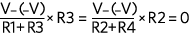When a single variable-resistive element (R3) changes by ΔR (R3 + ΔR):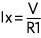1 × x(R3 + ΔR) = (-V) + (1 × x(-ΔR))

Since node A is at ground, the voltage at node B is: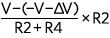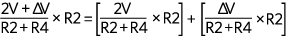We know thatterm is = 0. Hence the voltage at node B isAnd in a balanced bridge:The same equation can be written as:When using a balanced bridge with ratio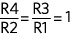, and we know that ΔV = Ix × ΔR and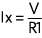, then the equation becomes:And simplistically putting R1 = R2 = R3 = R4 = R, then:At the output of the noninverting op amp, the equation then is:This suggests that the output from the second op amp is inverting in nature.

Figure 5 shows the transfer function and its nonlinearity from the Figure 4 implementation.In Figure 5, the linearity error, the absolute deviation from an ideal straight line, is less than 0.02%. When there’s improvement in absolute nonlinearity, there will also be improvement in the full-scale error (relative error).

Since there aren’t interacting resistive branches, you don’t have to apply precision matching of resistors. Rx and Rg variation will only yield a gain error, which can be calibrated at the same as the RTD device.

Based on the above data, it appears that this approach can be viable for 12-, 14-, 16-, and even 18-bit applications. It’s a simple design and requires very little calibration by the microcontroller. As noted earlier, this circuit is well established and has been widely used in the field for many years.

A dual-supply voltage for the front-end is needed to implement the circuit in Figure 4. This negative supply also requires additional board space and components; however, this may not be a viable option if this is the only place in the entire system where the negative supply is needed. A high-precision bridge sensor also needs low-offset voltage, low-offset drift, and low-noise performance.

### Using a Dual Op Amp for the Bridge Design

Consider this scenario: a single power supply for the amplifier used in Figure 4. As an example, let’s take a look at Maxim’s MAX44267, which operates from a single supply and can output bipolar voltages. Unlike other single-supply amplifiers requiring headroom above ground, the MAX44267 provides a true-zero output, which makes it ideal for bridge sensors (Figure 6). The MAX44267 integrates charge-pump circuitry that generates the negative voltage rail in conjunction with external capacitors. The amplifier can then operate from a single +4.5V to +15V power supply, while remaining as effective as a normal dual-rail ±4.5V to ±15V amplifier.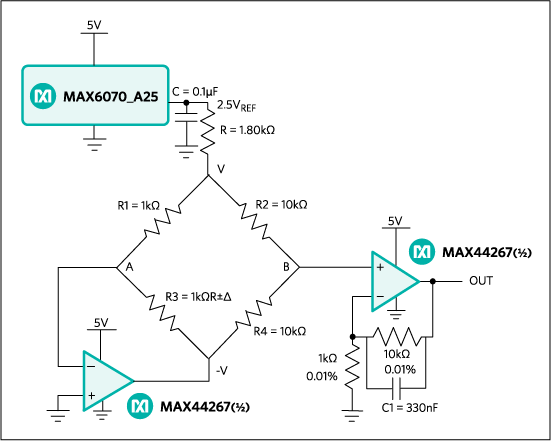In the Figure 6 circuit, you can implement the MAX44267 with a single supply voltage (positive supply, VCC). The integrated negative VSS generator or charge pump generates a negative supply voltage. One of the advantages of this architecture is that it eliminates the need for negative supply regulators while also reducing board layout space and cost.

Figure 7 includes the MAX6070_A25 voltage reference to generate a 2.5VOUT reference. A dual op amp (again, the MAX44267) is used with the resistance bridge where R1 = R3 = 1kΩ and R2 = R4 = 10kΩ. . To reduce the amount of current flowing through the bridge along with the power dissipation, an additional 1.8kΩ is used in series. At a balanced condition, the V(+) node becomes one-third the voltage reference’s output. This is followed with second-stage amplification with a gain of 11 at the OUTA node.In our examination, R3 was replaced by a Fluke® RTD calibrator, which served as the temperature-dependent resistance element (as PT1000) to evaluate a temperature-dependent change from -50°C to +155°C. For the given temperature change using a PT1000, the change in resistance (±R) is about 800Ω and an equivalent range of 325mV is effected (see Equation 4). Amplifier 2, since it boasts an internal negative supply, can accommodate this swing (-242mV to -83mV) at its input below ground, while providing an output gain of 11.

FIn the second stage of Figure 7, a Sallen-Key filter filters the input signal to the required bandwidth (in this instance, 50Hz). Without calibration or trim, we’re able to achieve full-scale error accuracy within ±0.05% is obtained from the bridge output at node B. This approach renders the transfer function of the bridge circuit linear. Using the MAX44267 results in improved front-end circuit performance.

### Test Measurements

1. Figure 8 shows the absolute bridge voltage output versus change in resistance (a linearity curve output), under 0.02%.
2.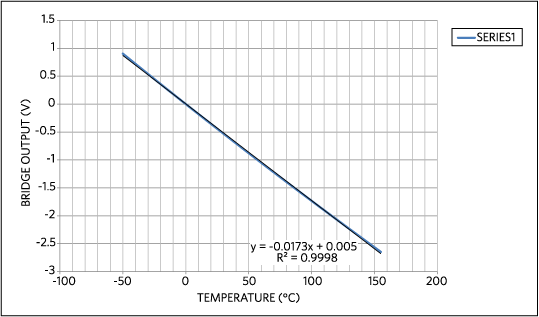3. Figure 9 shows the gain error plot as percentage versus full scale. The error curve shows small wriggles, in order of 0.002%, that are a combination of manual data plotting and measurement setup noise.
4.5. Figure 10 shows the voltage noise density of the bridge plus amplifier: 115nV/√ Hz at 1kHz and 500nV/√ Hz under 50Hz. A 50 Hz filter implemented in the second stage to removes the sensitivity to line noise.
6.7. Figure 11 shows the voltage noise(VP-P) of the bridge plus amplifier, 0.1Hz to 10Hz, 6µVP-P.
8.### 参考資料

1. Reference Data for Radio Engineers , Howard W. Sams & Co.inc (ITT).
2. Practical Design Techniques for Sensor Signal Conditioning, Section 2: Bridge Circuits by Walter Kester.
3. Wheatstone Bridge Nonlinearity, Measurements Group Tech Note TN-507, 12/18/2000, http://www.thermofisher.com.au/Uploads/file/Environmental-Industrial/Process-Monitoring-Industrial-Instruments/Sound-Vibration-Stress-Monitoring/Stress-Analysis/VishayMM/technology/technotes/TN-507-Wheatstone-Bridge-Nonlinearity.pdf.

A similar version of this application note appeared in Electronics 360 on June 23, 2015.